# 贝塞尔曲线在前端，走近她，然后爱上她

## 贝塞尔曲线在前端

css3的动画主要是

• transition
• animation

transition有`transition-timing-function`
animation有`animation-timing-function`

`transition-timing-function`为例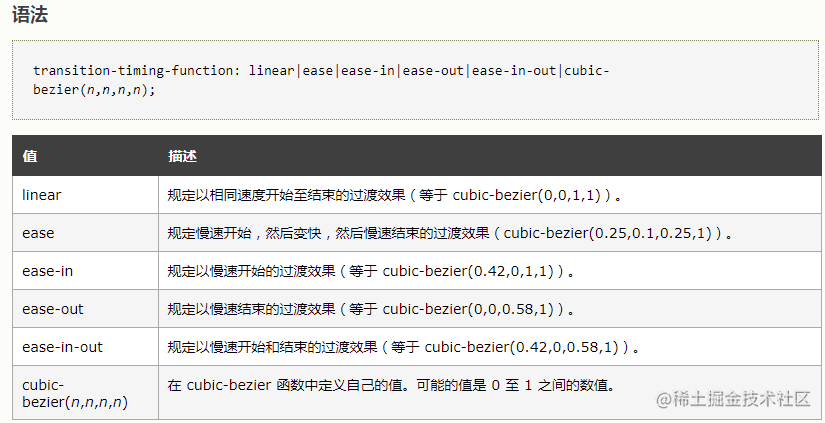1. 曲线越陡峭，速度越快，反之，速度越慢！
2. 控制点的位置会影响曲线形状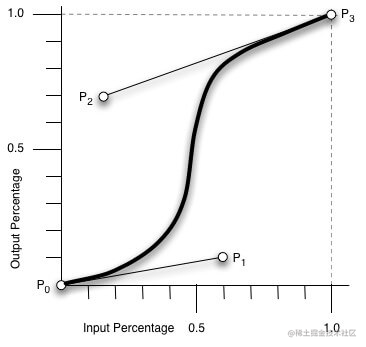• `svg`
• `canvas/webgl`
• `css3` 动画
• `animation Web API`
千万别以为JS就不能操作CSS3动画了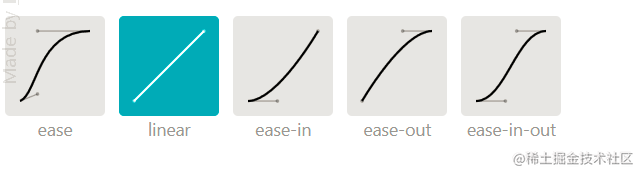## 什么是贝赛尔曲线

• P0的坐标(0,0), 最终的点的坐标为(1,1)

`t`从0不断的增长到1
`t`的值和控制点的x坐标套入公式，得到一个新的x坐标值
`t`的值和控制点的y坐标套入公式，得到一个新的y坐标值

(新的x坐标值 , 新的y坐标值)坐标就是`t`时刻曲线的点的坐标。

### 通用公式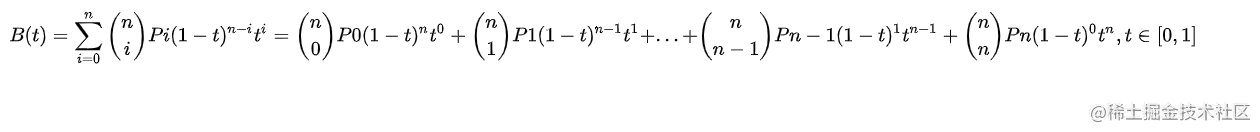### 线性公式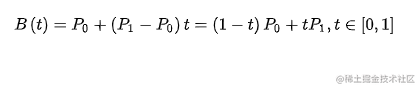### 二次方公式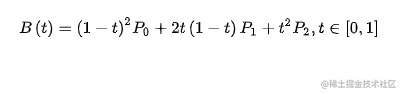### 三次方公式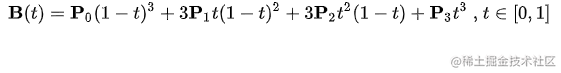P0作为起点，P3作为终点， 控制点是P1与P2, 因为我们一般会假定 P0 为 (0,0), 而 P3为(1,1)。

## 一阶二阶三阶封装

``````class Bezier {
getPoints(count = 100, ...points) {
const len = points.length;
if (len < 2 || len > 4) {
throw new Error("参数points的长度应该大于等于2小于5");
}
const fn =
len === 2
? this.firstOrder
: len === 3
? this.secondOrder
: this.thirdOrder;
const retPoints = [];
for (let i = 0; i < count; i++) {
retPoints.push(fn.call(null, i / count, ...points));
}
return retPoints;
}

firstOrder(t, p0, p1) {
const { x: x0, y: y0 } = p0;
const { x: x1, y: y1 } = p1;
const x = (x1 - x0) * t;
const y = (y1 - y0) * t;
return { x, y };
}

secondOrder(t, p0, p1, p2) {
const { x: x0, y: y0 } = p0;
const { x: x1, y: y1 } = p1;
const { x: x2, x: y2 } = p2;
const x = (1 - t) * (1 - t) * x0 + 2 * t * (1 - t) * x1 + t * t * x2;
const y = (1 - t) * (1 - t) * y0 + 2 * t * (1 - t) * y1 + t * t * y2;
return { x, y };
}

thirdOrder(t, p0, p1, p2, p3) {
const { x: x0, y: y0 } = p0;
const { x: x1, y: y1 } = p1;
const { x: x2, y: y2 } = p2;
const { x: x3, y: y3 } = p3;
let x =
x0 * Math.pow(1 - t, 3) +
3 * x1 * t * (1 - t) * (1 - t) +
3 * x2 * t * t * (1 - t) +
x3 * t * t * t;
let y =
y0 * (1 - t) * (1 - t) * (1 - t) +
3 * y1 * t * (1 - t) * (1 - t) +
3 * y2 * t * t * (1 - t) +
y3 * t * t * t;
return { x, y };
}
}

export default new Bezier();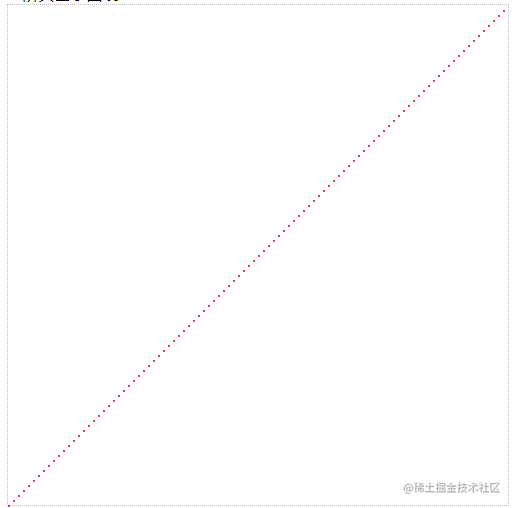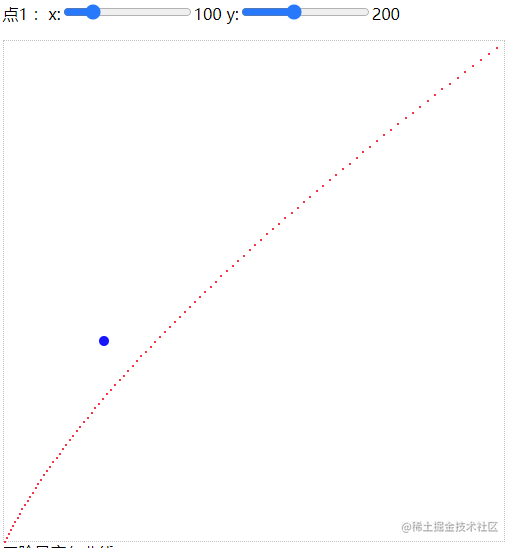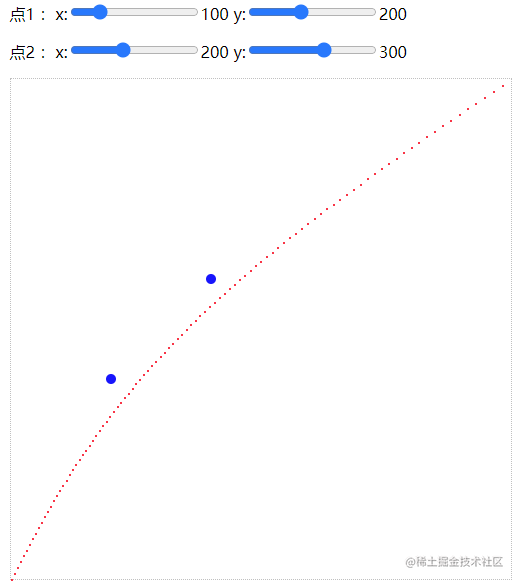## 贝塞尔曲线控制点

• canvas 绘制效果
canvas有`bezierCurveTo`方法，直接可以绘制贝塞尔曲线
• 两个控制点用dom元素来显示

• 点击时计算最近的点，同时修改最近点的坐标
• 重绘